# Training a Pytorch Lightning MNIST GAN on Google Colab

Marton Trencseni - Sat 20 February 2021 - Data

## Getting started with Google Colab

Google Colab is a free Jupyter-like service from Google. The easiest way to get started is to open the notebook for this post, and then click File > Save a copy in Drive, this will make a copy for you. Alternatively, in Google Drive, you can click New > More > Google Colaboratory to start with a blank notebook. Try it out, it's free, you get GPU training for free, and the Colab UI is very nice.

It's important to know that there are limits on Colab, it's free after all. After one hour of UI inactivity, the underlying Virtual Machine (VM) will shut down, and all memory/state and files you wrote to the VM's disk will be lost. You can re-run the notebook, but it will be on a fresh new VM. So when working on Colab, always make sure your notebook is complete, so that Runtime > Restart and run all results in correct execution. Also, even if you're active continuosly, the VM will be shut down after 12 hours. This model is good enough for experimentation if you don't hve access to your own GPU(s), but not for more serious work.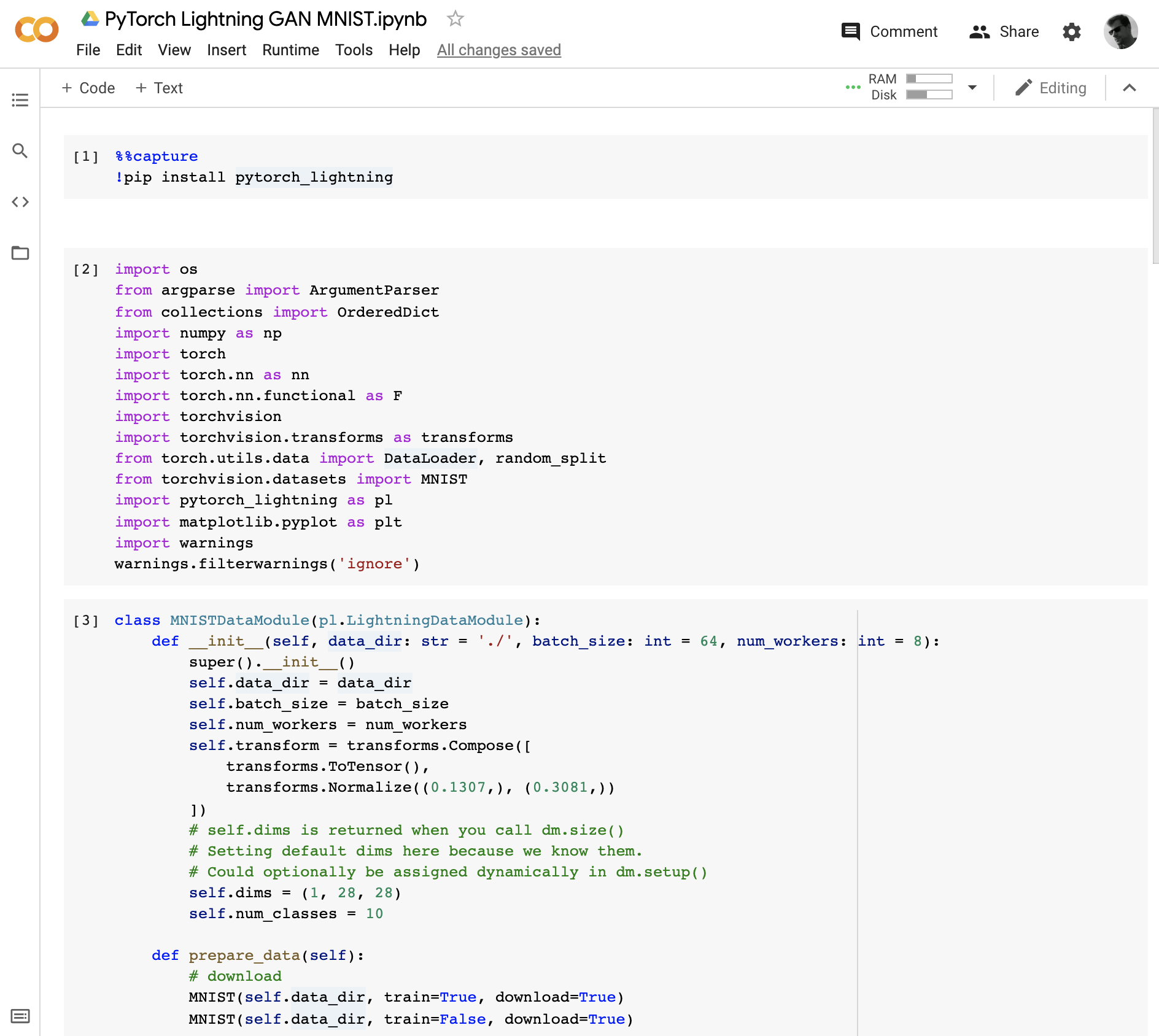## Enabling the GPU on Colab

In Colab, you get a notebook running on a VM with a GPU — but first you have to enable the GPU. Go to Edit > Notebook settings, and enable a GPU or a TPU. The GPU option is faster, go with that. Without this, the runtime will not see any GPUs! However, you only have to do this once, not on every VM restart.## GAN training with Pytorch Lightning

I will use one of Erik Linder-Noren's GANs called Softmax GAN. Erik's version is for Pytorch, and it was adapted for Pytorch Lightning and made an official Pytorch Lightning example.

What is Pytorch Lightning? It's a small framework on top of Pytorch that is quickly becoming the new standard. It helps you write more modular code by forcing you to factor out code into classes and callbacks. Usually, factoring existing Pytorch code into Lightning code is a simple matter, and results in less code (because Lightning has built in Trainers) and cleaner code. Check the official page for a quick explanation.

Since we're using MNIST here, note these previous Bytepawn posts about MNIST:

From Wikipedia:

The generative network generates candidates while the discriminative network evaluates them. The contest operates in terms of data distributions. Typically, the generative network learns to map from a latent space to a data distribution of interest, while the discriminative network distinguishes candidates produced by the generator from the true data distribution. The generative network's training objective is to increase the error rate of the discriminative network (i.e., "fool" the discriminator network by producing novel candidates that the discriminator thinks are not synthesized (are part of the true data distribution)).

A known dataset serves as the initial training data for the discriminator. Training it involves presenting it with samples from the training dataset, until it achieves acceptable accuracy. The generator trains based on whether it succeeds in fooling the discriminator. Typically the generator is seeded with randomized input that is sampled from a predefined latent space (e.g. a multivariate normal distribution). Thereafter, candidates synthesized by the generator are evaluated by the discriminator. Independent backpropagation procedures are applied to both networks so that the generator produces better images, while the discriminator becomes more skilled at flagging synthetic images. The generator is typically a deconvolutional neural network, and the discriminator is a convolutional neural network.

GANs often suffer from a "mode collapse" where they fail to generalize properly, missing entire modes from the input data. For example, a GAN trained on the MNIST dataset containing many samples of each digit, might nevertheless timidly omit a subset of the digits from its output. Some researchers perceive the root problem to be a weak discriminative network that fails to notice the pattern of omission, while others assign blame to a bad choice of objective function.

## Code

Let's start with the code for the Generator, a deconvolutional neural network (it produces an image from a lower dimensional [random] input):

class Generator(nn.Module):
def __init__(self, latent_dim, img_shape):
super().__init__()
self.img_shape = img_shape

def block(in_feat, out_feat, normalize=True):
layers = [nn.Linear(in_feat, out_feat)]
if normalize:
layers.append(nn.BatchNorm1d(out_feat, 0.8))
layers.append(nn.LeakyReLU(0.2, inplace=True))
return layers
self.model = nn.Sequential(
*block(latent_dim, 128, normalize=False),
*block(128, 256),
*block(256, 512),
*block(512, 1024),
nn.Linear(1024, int(np.prod(img_shape))),
nn.Tanh()
)

def forward(self, z):
img = self.model(z)
img = img.view(img.size(0), *self.img_shape)
return img


Next, the Discriminator, a convolutional neural network (takes an image and emits a binary True/False, whether the image is coming from the training set or not):

class Discriminator(nn.Module):
def __init__(self, img_shape):
super().__init__()

self.model = nn.Sequential(
nn.Linear(int(np.prod(img_shape)), 512),
nn.LeakyReLU(0.2, inplace=True),
nn.Linear(512, 256),
nn.LeakyReLU(0.2, inplace=True),
nn.Linear(256, 1),
nn.Sigmoid(),
)

def forward(self, img):
img_flat = img.view(img.size(0), -1)
validity = self.model(img_flat)
return validity


I will not reproduce the rest of the code here or discuss the detail of the Softmax GAN architecture, check the paper if you're interested.

## Running the code in Colab

Colab already comes with Pytorch pre-installed. The only library we have to install manually for this example to run is Pytorch Lightning:

# suppress boring pip output with capture
%%capture
!pip install pytorch_lightning


To see example images produces by the GAN I wrote this helper function, which is called after training completes:

def show_sample(model):
z = model.validation_z.type_as(model.generator.model.weight)
sample_imgs = model(z)
grid = torchvision.utils.make_grid(sample_imgs, nrow=10)
plt.figure(figsize = (10, 10))
plt.imshow(np.transpose(grid.detach().numpy(), (1, 2 ,0)), interpolation='nearest')


## Results

I've run the GAN varying the number of epochs, to see how the Generator improves over time. For lower epochs, I've run it multiple times to see variations. Each image shows 100 "randomly" generated [by the Generator network] digits.

num_epochs = 1, showing output from 3 different training runs: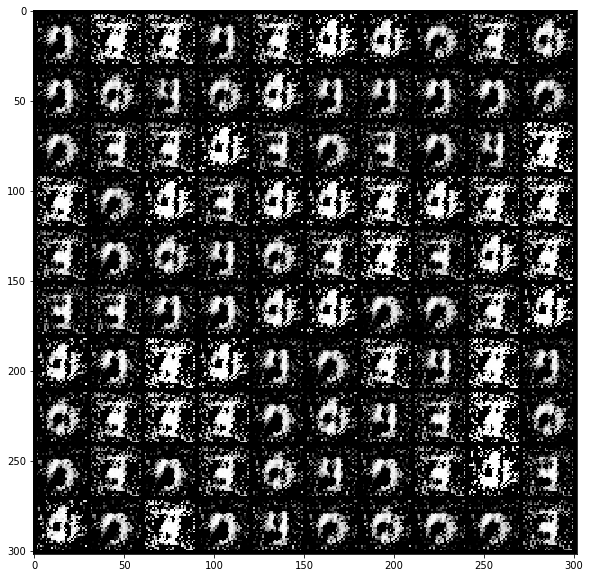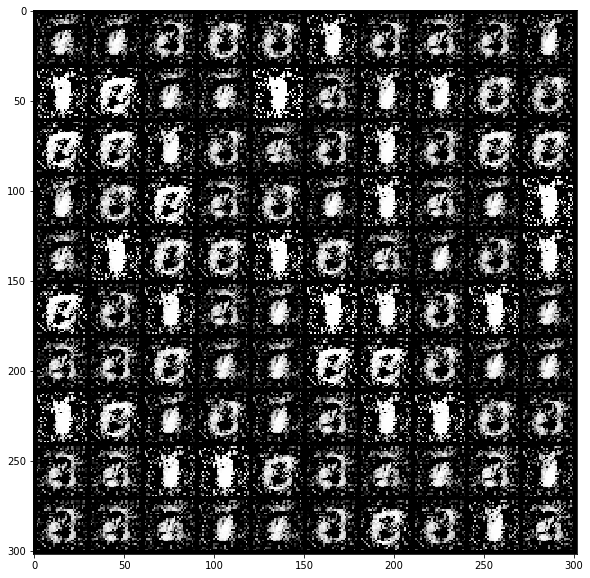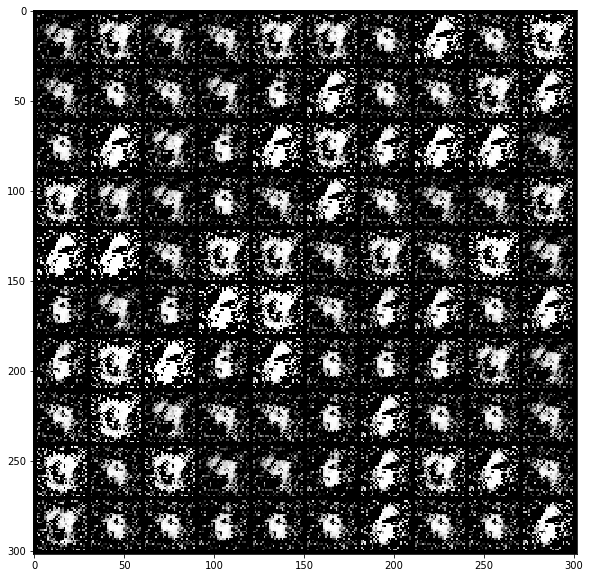num_epochs = 5, showing output from 3 different training runs: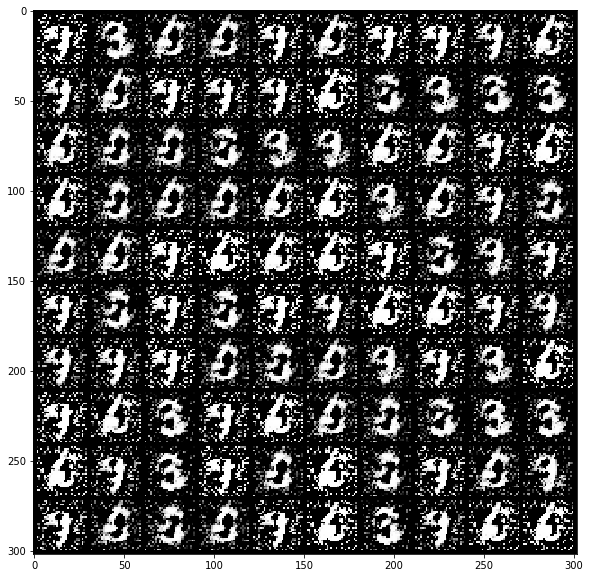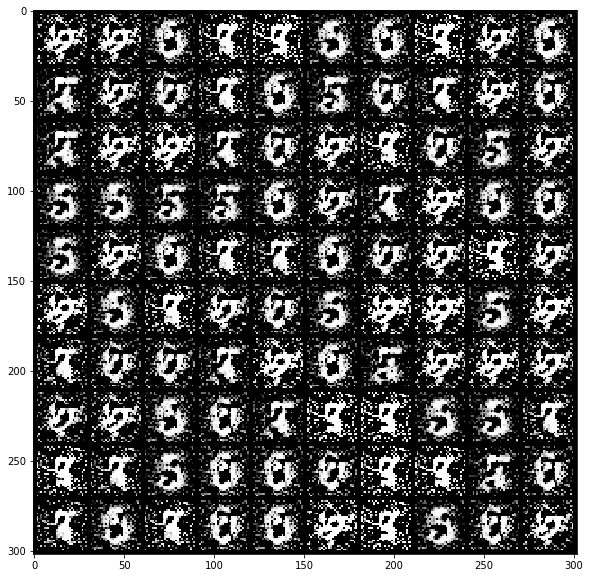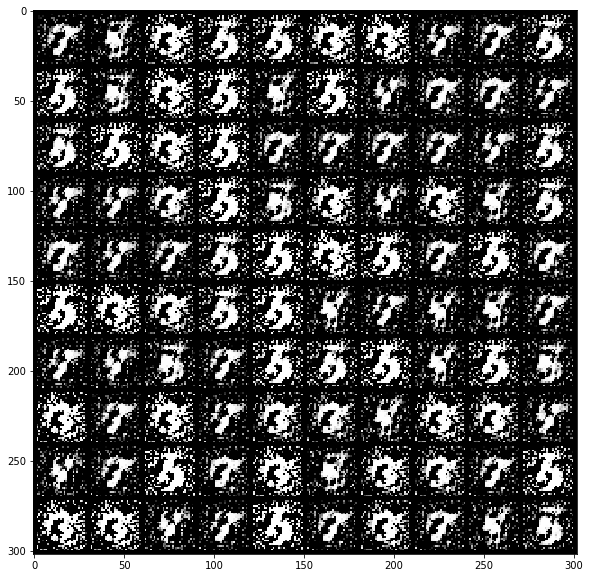num_epochs = 10, showing output from 3 different training runs: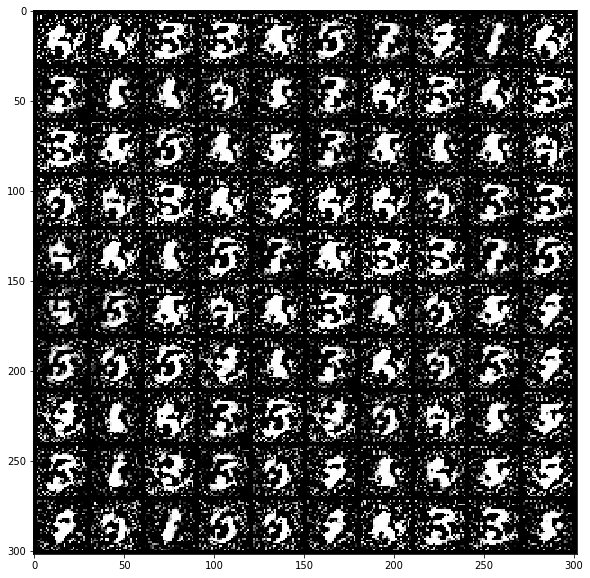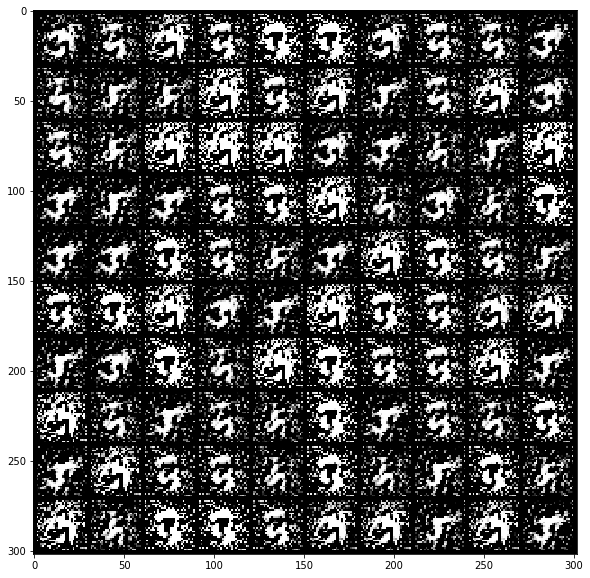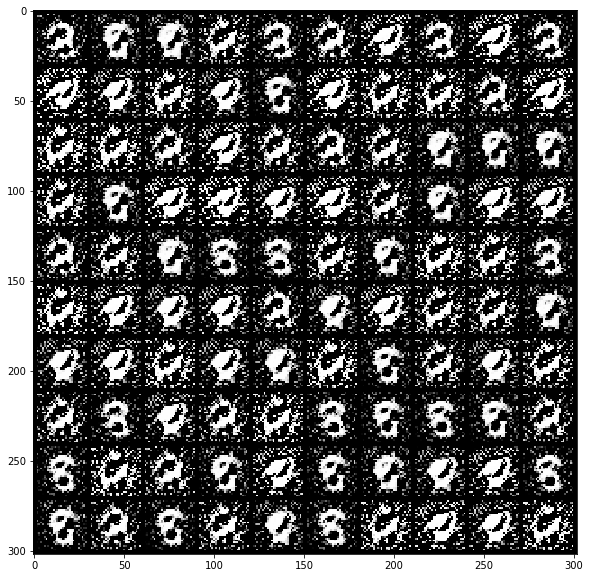num_epochs = 100, showing output from 1 training run: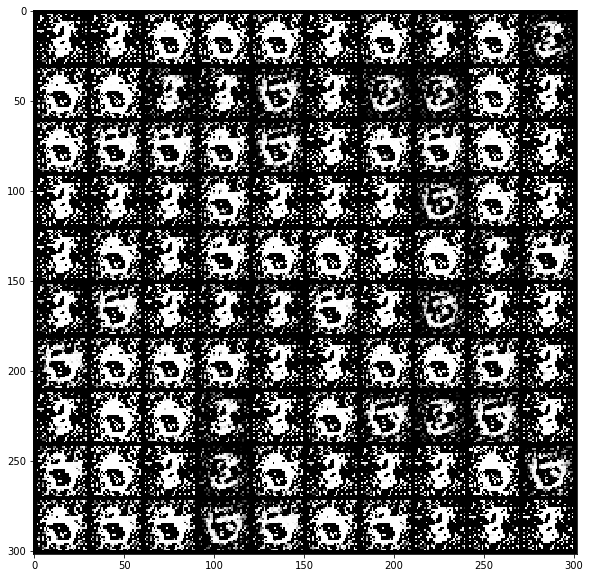num_epochs = 200, showing output from 1 training run: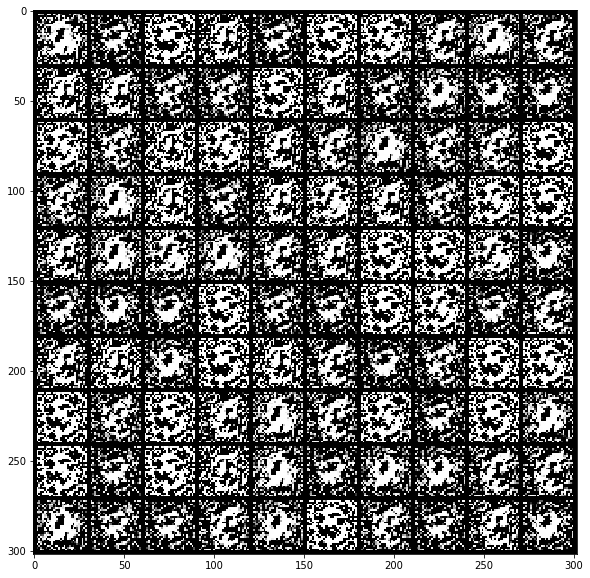## Conclusion

The results support the Wikipedia quote's "mode collapse" paragraph. The Generator tends to emit 1 or 2 digits. On the 4th image, it seems to be 5s and 7s, on the next 8s and 9s. The GAN does not converge to a good solution. In fact, I am having trouble visually seeing obvious benefits of training beyond 5 epochs. The other give-away is that the network produces too much noise. Any human could easily distinguish these from the MNIST digits, because those have no noise. Having said that, this could be solved easily by de-noising the resulting images using a traditional de-noising filter. I think with that the digits would be reasonably good.

Google Colab is a nice free notebook product from Google, and it's better than training on your laptop without a GPU. But even with a GPU, training for 100s of epochs is slow and is impractical for experimentation. In a subsequent post I will move training to AWS Sagemaker, where higher GPU instances are available, though not for free. With quicker training, I will try to fix the GAN to produce good quality images, like in this post.